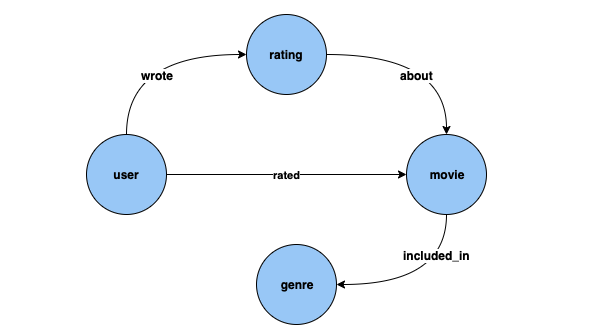Gremlin inference queries in Neptune ML - Amazon Neptune
Services or capabilities described in Amazon Web Services documentation might vary by Region. To see the differences applicable to the China Regions, see Getting Started with Amazon Web Services in China.

# Gremlin inference queries in Neptune ML

As described in Neptune ML capabilities, Neptune ML supports training models that can do the following kinds of inference tasks:

• Node classification   –   Predicts the categorical feature of a vertex property.

• Node regression   –   Predicts a numerical property of a vertex.

• Edge classification   –   Predicts the categorical feature of an edge property.

• Edge regression   –   Predicts a numerical property of an edge.

• Link prediction   –   Predicts destination nodes given a source node and outgoing edge, or source nodes given a destination node and incoming edge.

We can illustrate these different tasks with examples that use the MovieLens 100k dataset provided by GroupLens Research. This dataset consists of movies, users, and ratings of the movies by the users, from which we've created a property graph like this:Node classification: In the dataset above, `Genre` is a vertex type which is connected to vertex type `Movie` by edge `included_in`. However, if we tweak the dataset to make `Genre` a categorical feature for vertex type `Movie`, then the problem of inferring `Genre` for new movies added to our knowledge graph can be solved using node classification models.

Node regression: If we consider the vertex type `Rating`, which has properties like `timestamp` and `score`, then the problem of inferring the numerical value `Score` for a `Rating` can be solved using node regression models.

Edge classification: Similarly, for a `Rated` edge, if we have a property `Scale` that can have one of the values, `Love`, `Like`, `Dislike`, `Neutral`, `Hate`, then the problem of inferring `Scale` for the `Rated` edge for new movies/ratings can be solved using edge classification models.

Edge regression: Similarly, for the same `Rated` edge, if we have a property `Score` that holds a numerical value for the rating, then this can be inferred from edge regression models.

Link prediction: Problems like, find the top ten users who are most likely to rate a given movie, or find the top ten Movies that a given user is most likely to rate, falls under link prediction.